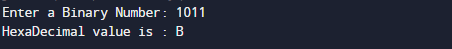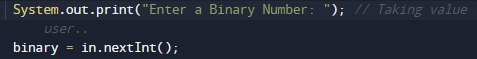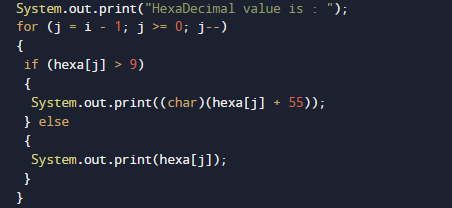# Java Program to Convert Binary to HexaDecimal

In this tutorial you will learn about the Java Program to Convert Binary to HexaDecimal and its application with practical example.

In this tutorial, we will learn to create a Java program that will Convert Binary to Hexadecimal  using Java programming language.

## Prerequisites

Before starting with this tutorial we assume that you are best aware of the following Java programming topics:

• Java Operators.
• Basic Input and Output
• Class and Object.
• Basic Java programming.
• Loops.
• Array.
• User Define Functions

## What Is Binary Number?

Number system where numbers are represented by using only two digits (0 and 1) with a base 2 is called a binary number system.

Hexadecimal number system is a  number system that has base as 16 i.e (hexa = 6 and deci = 10).Here  starting 10 value represent decimal number system and afterward start from A ,B to F in the hexadecimal system.Digits of the hexadecimal number system are 1, 2, 3, 4, 5, 6, 7, 8, 9, A, B, C, D, E, F.

## Java Program to Convert Binary to Hexadecimal.

In this blog we will create a program that will Convert Binary  to Hexadecimal number system.We would first declared and initialized the required variables.Then we would prompt user to input value in Binary and then we convert into Hexadecimal.

## OutputIn the above program, we have first declared and initialized a set variables required in the program.

• hexa = it will hold given binary number.
• decimal=  User define function.
• bianry= catch value passing through function calling.
• i&j= for iteration.
• Array.
• rem= hold the remainder.

In the next statement user will be prompt to enter a Binary number which will be stored in variable binary.## Algorithm :

1. First of all  we take a  binary number from user and convert into a decimal number.
2. First of all we need to convert the given binary number to decimal number, we extract each digit using the remainder method by dividing by 10.Than by multiplying the number digit with increasing powers of 2(Two).1. Process will continues until we reach the last digit  in each iteration.
2. After getting decimal number, just use for loop to get the desired output.As you can see use above for loop to print required output.

In this tutorial we have learn about the Java Program to Convert Binary to HexaDecimal and its application with practical example. I hope you will like this tutorial.